# Decimals on number lines#### Everything You Need in One Place

Homework problems? Exam preparation? Trying to grasp a concept or just brushing up the basics? Our extensive help & practice library have got you covered.#### Learn and Practice With Ease

Our proven video lessons ease you through problems quickly, and you get tonnes of friendly practice on questions that trip students up on tests and finals.#### Instant and Unlimited Help

Our personalized learning platform enables you to instantly find the exact walkthrough to your specific type of question. Activate unlimited help now!

0/1
##### Intros
###### Lessons
1. Introduction to Decimals on Number Lines:
How to create decimal number lines for decimal tenths and decimal hundredths
0/13
##### Examples
###### Lessons
1. Reading decimals on number lines
What is the decimal given by the point on the number line?

1.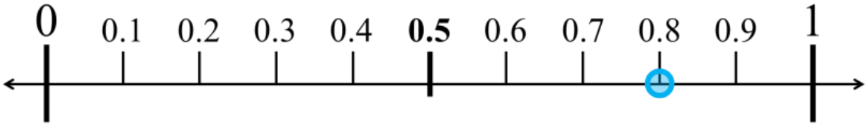2.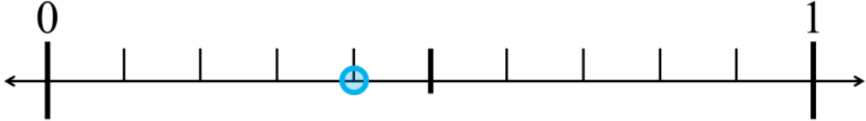3.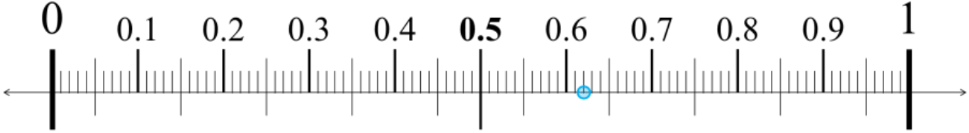4.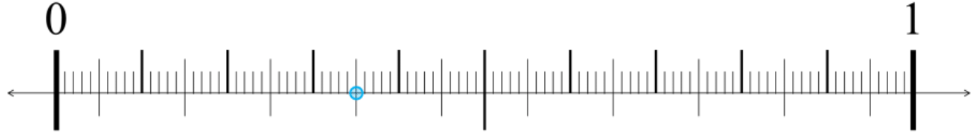2. Drawing decimals on number lines
Draw the decimal as a point on the number line

1.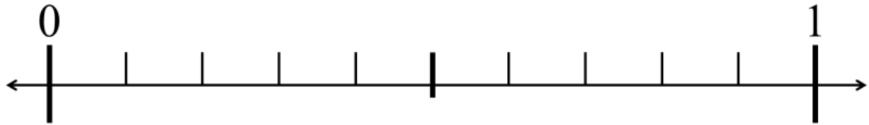2.3.4.3. Zooming into number lines and dividing into ten segments
Draw a point for the specified decimal. Label the number lines showing tenths and the zoomed in lines showing hundredths.

1.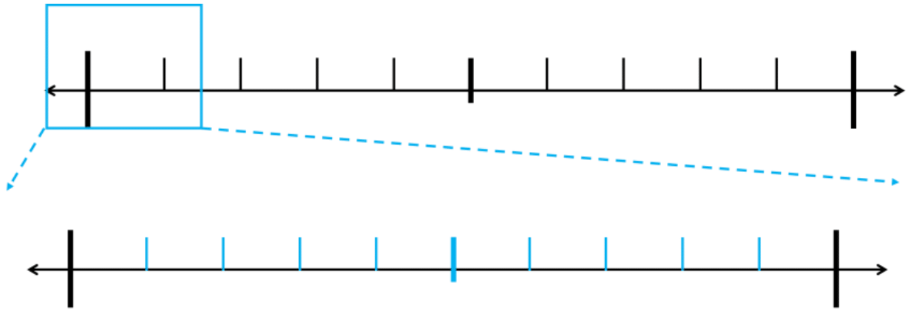2.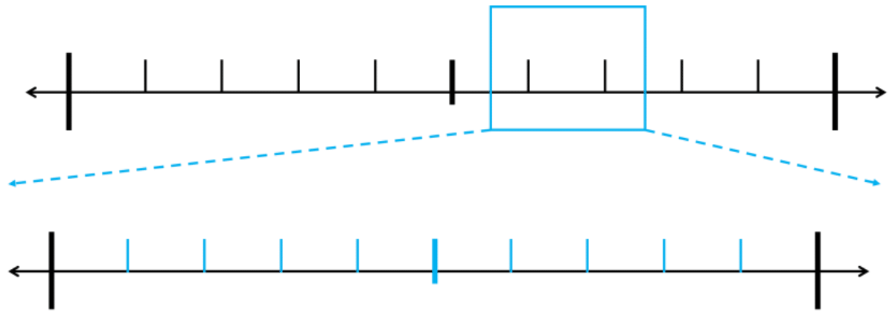3.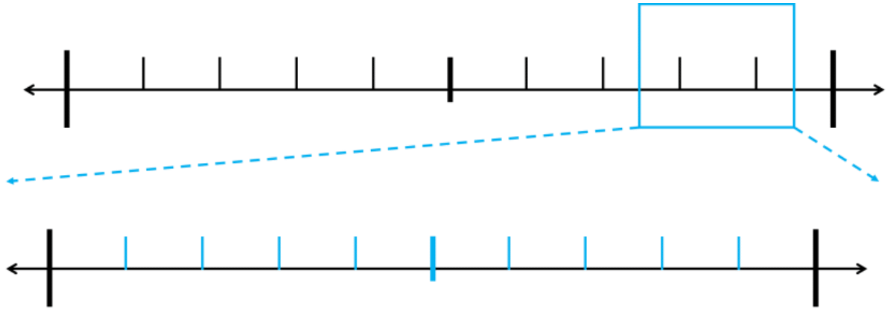4.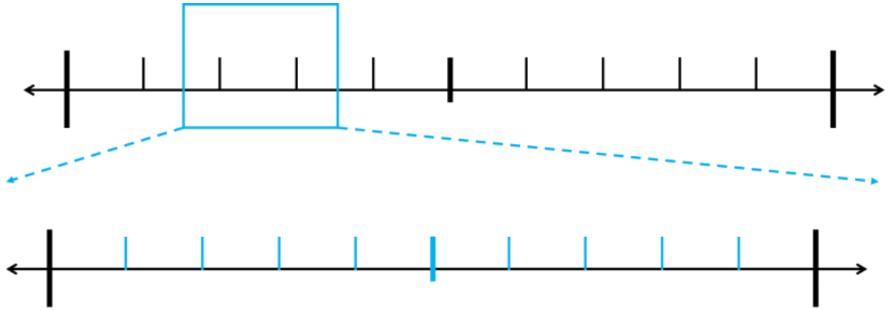5.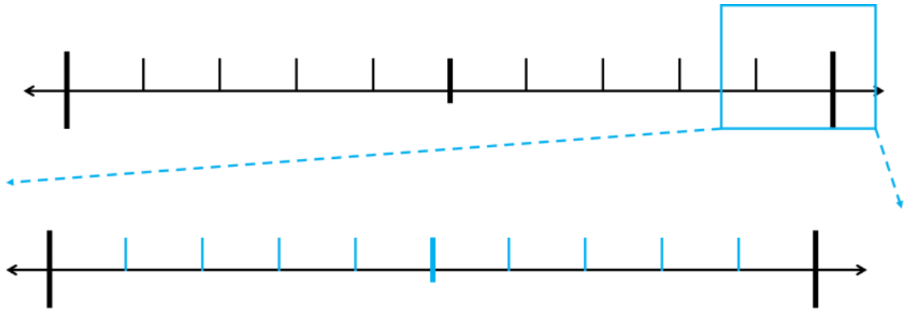0%
##### Practice
###### Topic Notes

In this lesson, we will learn:

• How to read number lines for decimals to the tenths or hundredths
• How to draw a point to represent a decimal on a decimal number line

Notes:

• A number line is a picture of a straight line with vertical markings of equal spacing
• Number lines increase from left to right (small $\, \longrightarrow \,$ big)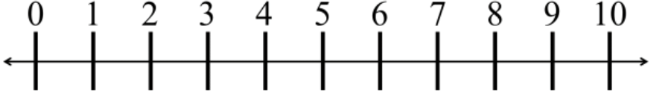• Decimals are numbers with place values even smaller than the ones place.
• Number lines can be divided even further between each number
• Splitting each division into 10 equal parts creates the next decimal place value
• Dividing the space between 0 to 1 into 10 equal parts, each part is a tenth
• Dividing the space between 0 to 0.1 into 10 equal parts, each part is a hundredth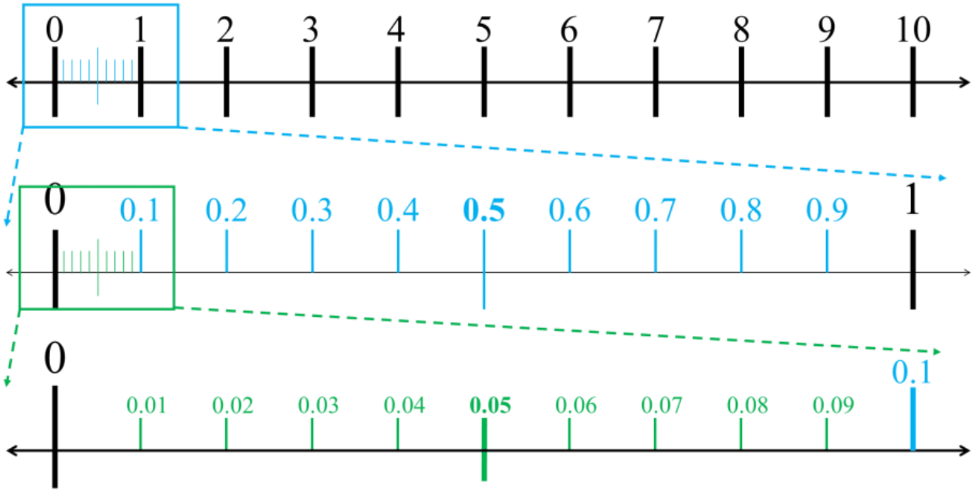• From 0 to 1, it is first split into 10 equal parts (tenths = 0.1)
• Each of these tenths 0.1 will be split into 10 more equal parts (10 tenths each into 10 parts = 10 × 10 = 100 parts)
• Ultimately, the space between 0 and 1 will be split into 100 equal parts
• That's why each 0.01 is called a hundredth (one of the 100 parts)